# The angle of lines

Calculate the angle of two lines y=x-21 and y=-2x+14

Result

α =  -108.4 °

#### Solution:Leave us a comment of example and its solution (i.e. if it is still somewhat unclear...):

Showing 0 comments:Be the first to comment!#### To solve this verbal math problem are needed these knowledge from mathematics:

For Basic calculations in analytic geometry is helpful line slope calculator. From coordinates of two points in the plane it calculate slope, normal and parametric line equation(s), slope, directional angle, direction vector, the length of segment, intersections the coordinate axes etc. Most natural application of trigonometry and trigonometric functions is a calculation of the triangles. Common and less common calculations of different types of triangles offers our triangle calculator. Word trigonometry comes from Greek and literally means triangle calculation.

## Next similar examples:

1. Angle between lines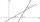Calculate the angle between these two lines: ? ?
2. Straight lines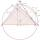Draw two lines c, d so that c || d. On line c mark points A, B, from point A start perpendicular to line c, from point B perpendicular to line c.
3. AngleA straight line p given by the equation ?. Calculate the size of angle in degrees between line p and y-axis.
4. SlopeFind the slope of the line: x=t and y=1+t.
5. Supplementary angles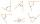One of the supplementary angles are three times larger than the other. What size is larger of supplementary angles?
6. Reference angleFind the reference angle of each angle:
7. Angles 1It is true neighboring angles have not common arm?
8. Obtuse angleWhich obtuse angle is creating clocks at 17:00?
9. LineIt is true that the lines that do not intersect are parallel?
10. Slope formFind the equation of a line given the point X(8, 1) and slope -2.8. Arrange your answer in the form y = ax + b, where a, b are the constants.
11. LineLine p passing through A[-10, 6] and has direction vector v=(3, 2). Is point B[7, 30] on the line p?
12. V - slopeThe slope of the line whose equation is -3x -9 = 0 is
13. Coordinates of vector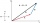Determine the coordinate of a vector u=CD if C(19;-7) and D(-16;-5)
14. Lie/do not lie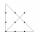The function is given by the rule f(x) = 8x+16. Determine whether point D[-1; 8] lies on this function. Solve graphically or numerically and give reasons for the your answer.
15. Perpendicular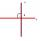Determine the slope of the line perpendicular to the line p: y = -x +4.
16. Profit gainIf 5% more is gained by selling an article for Rs. 350 than by selling it for Rs. 340, the cost of the article is:
17. Holidays - on poolChildren's tickets to the swimming pool stands x € for an adult is € 2 more expensive. There was m children in the swimming pool and adults three times less. How many euros make treasurer for pool entry?